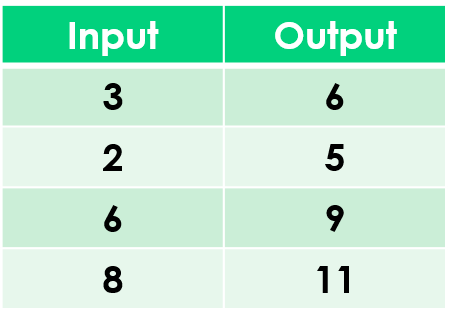1
visibility

Find the rule from the table below:• A

• B

Addition is the operation that increases a number by adding to it.

Solution:

To find the correct rule, we consider the given table.

We observe that the given rule makes each number increase. This implies addition.

We try adding 3 to each input number:

3 + 3 = 6
2 + 3 = 5
6 + 3 = 9
8 + 3 = 11

On adding 3 to the input numbers, we get the corresponding output numbers.

So, the rule is "add 3”.